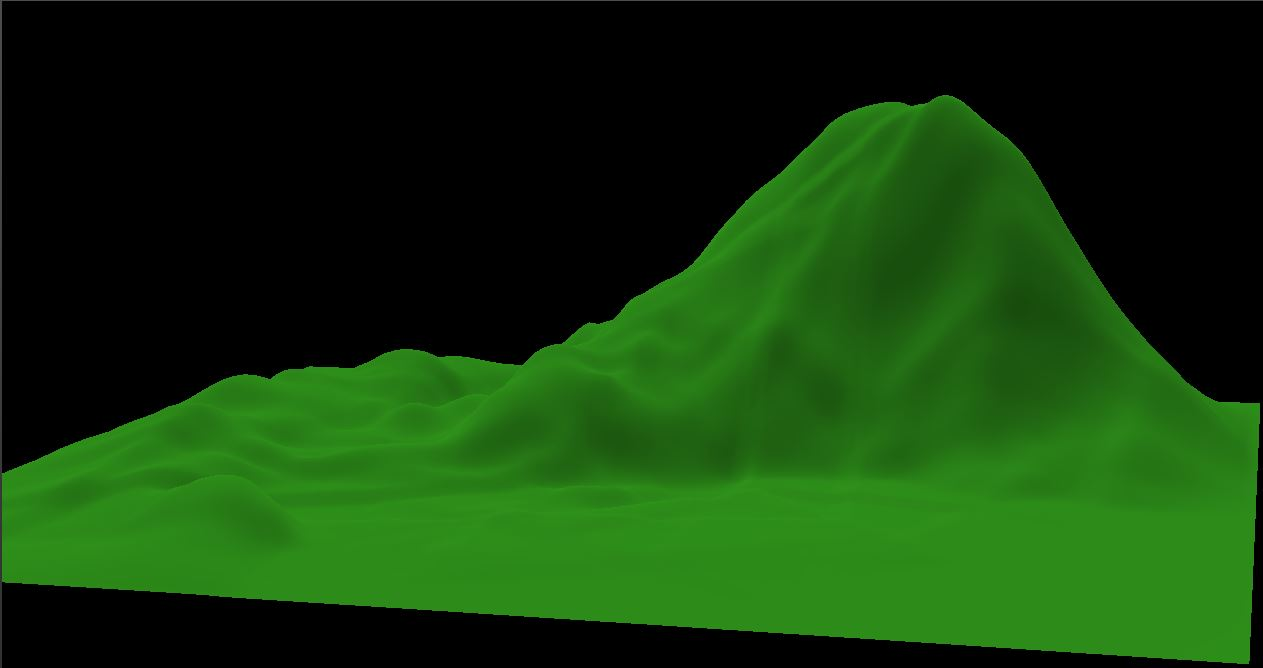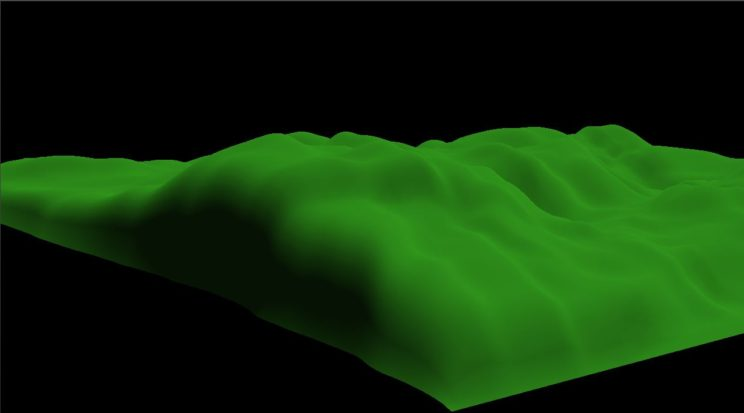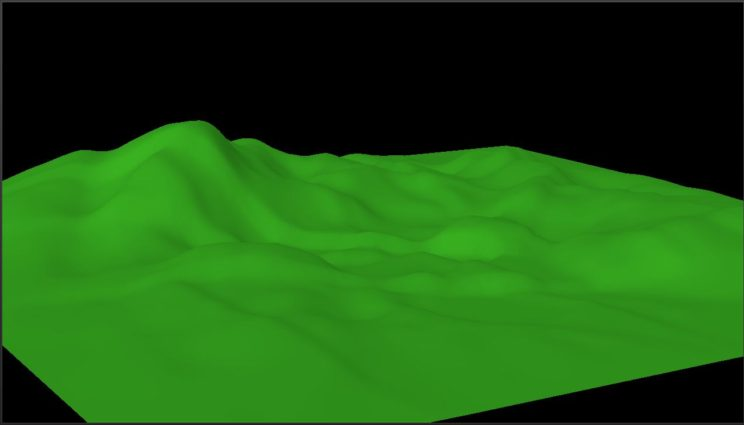# HoloLens Terrain Generation Demo Part 6 – Taking a Surface Into Account

I had a hard time with the title for this one. Basically, what I’m attempting to do is prepare the terrain generation algorithm to take into account that the height map will hopefully be attached to a flat horizontal surface in the final demo. This means we need to account for two limitations in the generator.

1. The hologram is resting on a surface in real life. If we have negative height values, parts of the hologram will not be visible since they will be rendered inside the real surface; therefore, we do not want our height map to ever go negative.
2. We want the hologram to be attached to the surface. For that to occur, the edges of the hologram’s mesh need to remain on the surface; therefore, the height values of the edge vertices must remain 0.

The first part can be dealt with with one line of code. Ensuring the height values don’t go below zero can be accomplished by simply setting a negative height value to 0.

`if (m_heightmap[y * (m_wHeightmap * m_resHeightmap + 1) + x] < 0) m_heightmap[y * (m_wHeightmap * m_resHeightmap + 1) + x] = 0;`

This doesn’t make a huge change to the terrain generated. As you can see in the following screen shot, there are some flat areas where previously there would have been a valley.

With that fixed, we can move on to locking the edges to zero.
It’s pretty easy to lock the edges to zero by simply ignoring them. Instead of running the loops that walk the height map from 0 to Num_Vertices_X – 1 like we were previously, we run the loops from 1 to Num_Vertices_X – 2 and likewise for the Y direction. This change needs to be made for both the IterateFaultFormation() and FIRFilter()1 methods.
The thing about doing this is that we don’t want to just say the edges are zero and let everything else continue to be modified the same way as always. If we do that, we’ll often wind up getting unnatural looking cliffs around the edge of the terrain.The solution I came up with to make something that more smoothly tapered in from the edges was to use the Manhattan distance of a given vertex from the center of the height map.
Let’s look at the case in the X direction. For the point p, we subtract p.x from half the width of the height map. If we take the absolute value of the result, that value will always be between 0 and half the width. If we divide that by half the width, we now have a value between 0 and 1. That value is currently reversed, however, with 1 on the edges and 0 in the middle. Thus, we need to subtract that value from 1 to get a scaling factor for the X direction. We can do the same for the Y direction. If we then multiply those two values together, we get a single scaling factor that we can multiply our height value for p by.

```float Terrain::CalcManhattanDistFromCenter(Windows::Foundation::Numerics::float2 p) {
unsigned int h = m_hHeightmap * m_resHeightmap + 1;
unsigned int w = m_wHeightmap * m_resHeightmap + 1;
float h2 = (float)h / 2.0f;
float w2 = (float)w / 2.0f;
float Dx = 1 - (abs(w2 - p.x) / w2);
float Dy = 1 - (abs(h2 - p.y) / h2);

return Dx * Dy;
}```

The result is flat terrain around the edges, sloping up into a circular island in the center. Unfortunately, the scaling factor significantly subdues the height values, resulting in a pretty low terrain compared to previous versions.

We’re currently scaling up linearly (or roughly so) from the edge to the center. What if we scale up from 0 to 1 faster, so that a greater portion of the height map is essentially unaffected by the scaling? To do so, we’ll simply change the return value of the above method.

`return min(Dx * Dy * 4.0f, 1.0f);`

The result is a much better looking terrain.This is giving me results I’m pretty happy with. The featured image at the top uses this version of the generator over 500 iterations. I think it looks pretty cool.

So what’s next? This is going to be the tough part. I need to figure out whether it is possible/how to find a real life surface and then attach the height map to the top of it. I’m pretty certain this is possible, since it looks like they’ve already done this with Minecraft, but we’ll see if I can figure it out. Jump to about 2:25 to see what I’m talking about.